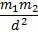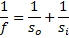### Category: Week 1 (06/11 - 06/17) Due June 17

05/28/2017
06/17/2017

Part 1:  Algebra (Rearrange)

1.  Using the equation vf = vo + at, solve for a

2.  Using the equation vf2 = vo2 + 2ax, solve for vo,

3.  Using the equation, F = G, solve for m2

4.  Using the equation, F = G, solve for d

5.  Using the equation., solve for so

Part 2:  Algebra (Plug and Chug)
6.  Using the equation vf = vo + at, solve for vf if vo is 15, a is 2.57, and t is 14.

7.  Using the equation vf2 = vo2 + 2ax, solve for vf if vo is 4.5, a is 3.87, and x is 15.

8.  Using the equation, F = G, solve for F if G is 6.67 x 10-11, m1 is 1.99 x 1030,
m2 is 1.90 x 1027, and d is 7.78 x 1011.

9.  Using the equation, F = G, solve for d if F = 3.5277 x 1022, G is 6.67 x 10-11
m1 is 1.99 x 1030, and m2 is 5.98 x 1024

10.  Using the equation., solve for f if so is 3.57, and si is 4.84

Part 3: Geometry/Trigonometry
(For Physics, make sure your calculator is set in degrees)

11.  As an angle increases from 0 to 90 degrees, how does the cosine of the angle change?
Prove. (show examples)

12.  As an angle increases from 0 to 90 degrees, how does the sine of the angle change?
Prove. (show examples)

13.  As an angle increases from 0 to 90 degrees, how does the tangent of the angle change?
Prove. (show examples)

14.  Why is there an error domain on your calculator for any inverse sine greater than 1?

15.  As an angle increases from 0 to 180 degrees, explain what happens to the tangent
values.  Prove.  (show examples)

Part 4:  Word Problems

speed = (distance / time) or speed x time = distance,  speed is a "rate"

Special Note:  WKP and WKS is the first letter of the authors last name of the textbook this problem was taken.

Need help:   check out:

16. Two cars leave the same place at the same time and travel in opposite directions,  one of the cars is traveling 10 miles per hour faster than the other car.   After 3 hours they are 240 miles apart. What is the speed of each car in miles per hour? (WKP, p56, #15)

17. Two planes which are 1060 miles apart leave at the same time and fly toward each other, meeting in 4 hours. If their speeds differ by 15 miles per hour,  what is the speed of each plane? (WKS, p56, #16)

18. A motorist drove to a certain city in 3 hours, and returned by another route which was 22 miles longer. On the return trip he traveled 10 miles per hour and it took him 2 hours and 50 minutes. What is the length of the shorter route? (WKS, p61,,#3)

19. Fred averaged 58 mph (miles per hour) when driving from his home to the Rose Bowl, and 50 mph when returning home on the same route. Find the distance along this route if his time returning was 1 hour 4 minutes longer than his time going to the Rose Bowl. (WKS, p120, #8)

20. A pilot has safe flying time of 88 minutes. How far east can she fly when there is no wind if her air speed going is 300 mph, and her speed returning is 250 mph?  (WKS, p120, #9)